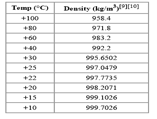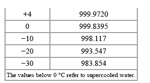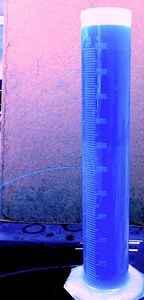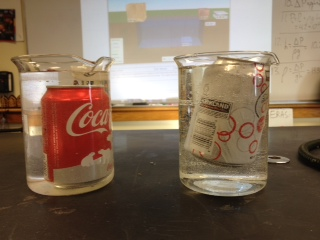# Fluids

The word fluid is a loaded word in physics. Both liquids and gases have similar physical behaviors, and thus when we talk about fluid dynamics we could be talking about either liquids or gases.  Terms such as pressure, buoyancy, Pascal’s principle, convection, Bernoulli’s principle work equally well for both states of matter.

# Liquid Density

Yesterday we looked at density.  A few thoughts when it comes to the density of liquids and here are a few examples from hyperphysics.

 Liquids Water at 4 C 1.0000 Water at 20 C 0.998 Gasoline 0.70 Mercury 13.6 Milk 1.03

Seawater is much denser than freshwater.  A good number to use for saltwater density is 1.04 g/ml, of course this number varies with salinity and temperature.  The density of the Dead Sea is 1.24 g/ml.

Most materials expand with increasing temperature and water is generally no exception as you can see from this table.But notice the strange behavior once water gets below 4 degrees Centigrade!  Instead of getting denser, water starts to expand.  That means that ice has a density less than slightly warmer water…thus it floats.  And as the ice gets colder it continues to expand for several more degrees. This is due to the VanDerWall forces.  This physics of ice is actually quite complex and we will discuss this more when we get to phase changes.

# Hydrostatic Pressure

Of course, all of this water has weight, and if you swim to the bottom of a swimming pool you can feel the pressure from the water pushing in on your ears.  If you have ever carried a bucket of water with a hole in it, you can attest to the pressure which pushes the water out.

This pressure only depends on the depth of the liquid and the density of the liquid (and the presence of gravity).

liquid pressure. P = weight density x depth

P(hydro) = P (atmos) + ρgΔh

P is for pressure, ρ is the density of the liquid, g is the acceleration due to gravity, and Δh is the depth of the liquid.  Note there are two Pressures in this equation.  The Pressure due to the atmosphere is on top of all that liquid too!  Above that 5 meters of water you have several miles of air on top of you.  So whenever you calculate hydrostatic pressure, you also have to add in the atmospheric pressure.

If we were just trying to find the change in pressure in a liquid we could ignore the atmosphere

ΔP = ρgΔh

To understand where this equation comes form you can think of the weight of a column of fluid with a height h, and cross sectional area A.  Thus the volume of the column of fluid is

V = A·h

The force of gravity on this column is

F = mg = ρVg = ρ A h g

where we use the definition of density as ρ = mV.

As we define pressure as force per unit area due to this column of fluid is

P = F/A = ρ A h g/ A = ρ g h

This PHET simulation is a great way to explore the role of hydrostatic pressure.  In this simulation you can vary density, depth, atmosphere, and gravity.

One cool example that you can model with the PHET simulation ishow far the water comes out of a tank, and how this varies with depth.  The deeper hole shoots water farther.  Why?

Another interesting things is what happens to an object at lower or higher pressures?  A SCUBA diver carries an inflatable bladder (balloon) to add extra buoyancy. As they diver deeper, the increased water pressure will compress the bladder.

Similarly, as the diver ascends, the bladder will expand.  Divers must be careful to exhale as the ascend as their lungs are balloons too! An example of this can be seen in this video of a marshmallow in a vacuum chamber.

Next time….what is happening in this picture?